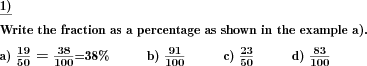Custom math worksheets at your fingertips# Details for problem "Fraction to percentage"

Quickname: 7407

Elementary School, Primary School, Junior High School, Middle School, High School.

## Summary

A fraction is given, write as a percentage.

## Example## Description

A fraction is bar notation is presented. The value of the fraction is always between 0 and 1, which relates to a percentage of 0 to 100%. The value of the fraction has to be written down in percentage notation.

The number of decimal places for the percentage value can be chosen.

The number of problems is selectable. As an option, the first problem can be shown with the answer to serve as an example.

Download free printable worksheets for this math problem here. The worksheet contains the problems only, the solution sheet includes the answers. Just click on the respective link.

•Worksheet 1Solution sheet with answers
•Worksheet 2Solution sheet with answers
•Worksheet 3Solution sheet with answers

If you can not see the solution sheets for download, they may be filtered out by an ad blocker that you may have installed. If this is the case, please allow ads for this page and reload the page. The solution sheets will then reappear.

• Do these sample worksheets do not really fit?
• Do you need more math worksheets, with a different level of difficulty?
• Would you like to combine different problems on a worksheet and adjust them to your needs?
• As a teacher, you can put together your own worksheets using the automatically generated math problems provided.
With a free initial credit, you can start creating your own math worksheets in a few minutes.

You can try it for free! Register here, to create custom worksheets now!

## Customization options for this problem

Parameter
Possible values
Number of problems
1, 2, 3, 4, 5, 6, 7, 8, 9, 10
Decimal places for percentage
0, 1, 2, 3, 0-1, 0-2, 0-3
Sample problem
Yes, No

## Similar problems

Remark
Description
The other way round - write as a fraction
A percentage has to be written in fraction bar notation.Deutsche Version dieser Aufgabe
These informational pages with samples describe math problems that can be combined on custom math worksheets with solutions for home and K-12 school use.
Deutsche Seiten
×